# Basic

Basic components for AC multiphase models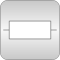# Package Contents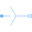Star Star connection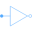Delta Delta (polygon) connection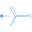MultiStar Star connection of multi phase systems consisting of multiple base systems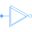MultiDelta Delta (polygon) connection of multi phase systems consisting of multiple base systems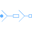MultiStarResistance Resistance connection of star points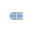PlugToPin_p Connect one (positive) pin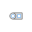PlugToPin_n Connect one (negative) pin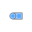PlugToPins_p Connect all (positive) pins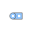PlugToPins_n Connect all (negative) pins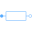Resistor Multiphase linear resistorConductor Multiphase linear conductor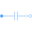Capacitor Multiphase linear capacitor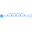Inductor Multiphase linear inductor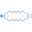MutualInductor Linear mutual inductor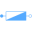Impedance Multiphase linear impedanceAdmittance Multiphase linear admittance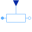VariableResistor Multiphase variable resistorVariableConductor Multiphase variable conductor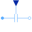VariableCapacitor Multiphase variable capacitor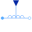VariableInductor Multiphase variable inductor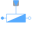VariableImpedance Multiphase variable impedanceVariableAdmittance Multiphase variable admittance

# Information

This information is part of the Modelica Standard Library maintained by the Modelica Association.

This package hosts basic models for quasi stationary multiphase circuits. Quasi stationary theory can be found in the references.